## Eigenvalue Problems with Matrices

It is often convenient to solve eigenvalue problems likeusing matrices. Many problems in Quantum Mechanics are solved by limiting the calculation to a finite, manageable, number of states, then finding the linear combinations which are the energy eigenstates. The calculation is simple in principle but large dimension matrices are difficult to work with by hand. Standard computer utilities are readily available to help solve this problem.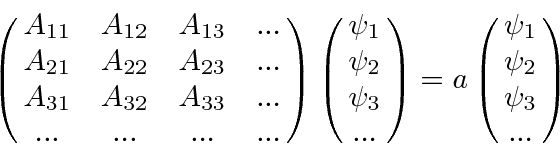Subtracting the right hand side of the equation, we haveFor the product to be zero, the determinant of the matrix must be zero. We solve this equation to get the eigenvalues.* Example: Eigenvectors of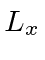.*

The eigenvectors computed in the above example show that the x axis is not really any different than the z axis. The eigenvalues are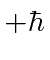,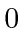, and, the same as for z. The normalized eigenvectors ofareThese vectors, and anyvectors, can be written in terms of the eigenvectors of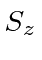.

We can check whether the eigenvectors are orthogonal, as they must be.The others will also prove orthogonal.

Shouldandbe orthogonal?
NO. They are eigenvectors of different hermitian operators.

The eigenvectors may be used to compute the probability or amplitude of a particular measurement. For example, if a particle is in a angular momentum state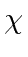and the angular momentum in the x direction is measured, the probability to measureisJim Branson 2013-04-22